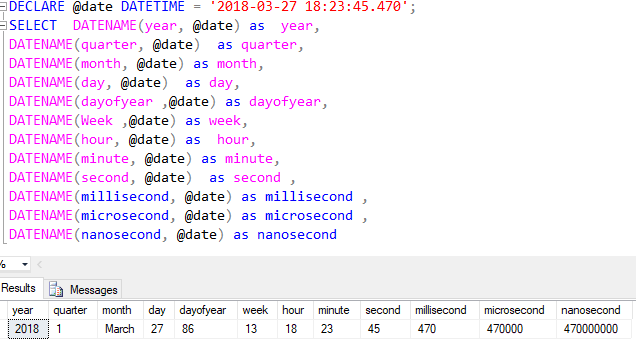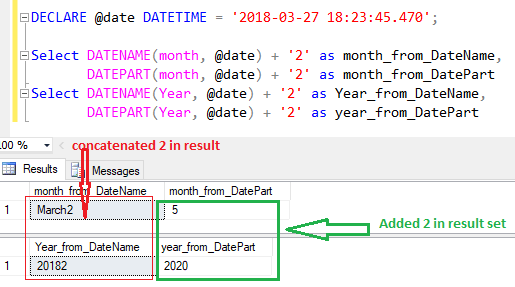Home » SQL DATENAME()

# SQL DATENAME()The DATENAME() function returns a string, navarchar type, that represents a specified date part of date, it can be a day, month, year or time of specific date.

DATENAME() is similar to theexcept for the return type.

The DATENAME() function returns the date part as a character string whereas the DATEPART() returns the date part as an integer.

SYNTAX

`DATENAME (datepart, inputdate) `

datepart is the part of a date to be extracted.

inputdate is the date from which the date part is extracted.

Lets look at an example of DATENAME function in SQL.

Following statement uses DATENAME Function, that extract the date parts from given date ‘2018-03-27 18:23:45.470’

```DECLARE @date DATETIME = ‘2018-03-27 18:23:45.470’;
SELECT DATENAME(year, @date) as  year,
DATENAME(quarter, @date)  as quarter,
DATENAME(month, @date) as month,
DATENAME(day, @date)  as day,
DATENAME(dayofyear ,@date) as dayofyear,
DATENAME(Week ,@date) as week,
DATENAME(hour, @date) as  hour,
DATENAME(minute, @date) as minute,
DATENAME(second, @date)  as second ,
DATENAME(millisecond, @date) as millisecond ,
DATENAME(microsecond, @date) as microsecond ,
DATENAME(nanosecond, @date) as nanosecond```As you can see, It returns all the part of date such as Quarter, Month, day, dayofyear, week, hour, minute, second, milisecond, microsecond, and nanosecond.

So using DATENAME function you can get any part of date.

Following are the valid datepart list that you can use to get a part of date as per your requirement.

```day                 d, dd
month               m, mm
year                yy, yyyy
quarter             qq, q
hour                hh
minute              mi, n
second              ss, s
millisecond         ms
microsecond         mcs
nanosecond          ns
week                wk, ww
dayofyear           dy, y

```

#### DATEPART VS DATENAME

Lets look at an example considering the difference between DATEPART and DATENAME.

DATEPART returns a part of date as an integer where as DATENAME returns of datatype string.

So if you add any number to result returned by DATEPART, it adds the number to result and returns the modified result where as when you add any number to the result returned by DATENAME. It concatenates the number with the result instead of adding it.

Lets look at an example, in following statement you can see for given date we try to add value 2 in the result returned by DATEPART and DATENAME function for the date part of month and year for given date.

You can see the final result where DATEPART function adds the 2 in result while DATENAME concatenates the 2 with result.

```DECLARE @date DATETIME = ‘2018-03-27 18:23:45.470’;

SELECT DATENAME(month, @date) + '2' as  month_from_DateName,

DATEPART(month, @date) + '2' as  month_from_DatePart

SELECT DATENAME(year, @date) + '2' as  year_from_DateName,

DATEPART(year, @date) + '2' as  year_from_DatePart,```